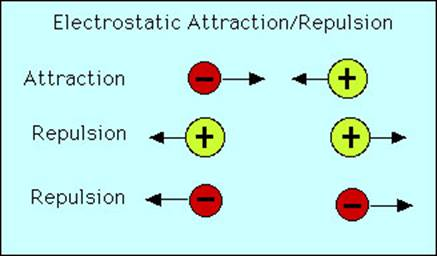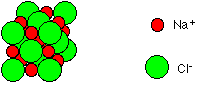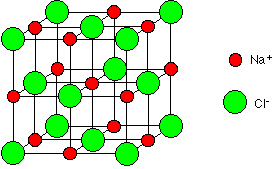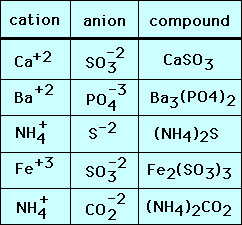Worksheet 6.3, 6.4 - Ions and Ionic Compounds

For group A representative elements, the group number gives the number of electrons in the outermost energy level.  These electrons are the ones that participate in chemical bonding and are called valency electrons.

1.  How many valency electrons do each of the following elements have?

a)  potassium                            b)  sulfur                       c)  nitrogen

d)  calcium                                e)  iodine                      f)  neon

An ion is a charged atom. If an atom loses electrons, it will have more protons than electrons and therefore have a positive charge forming a cation.  If an atom gains electrons, it will have more electrons than protons and therefore have a negative charge forming an anion.

Remember that metals tend to lose electrons and nonmetals to gain electrons to achieve the octet or 8 electrons in the outer energy level of a noble gas because this is very stable.  Metals will then lose their valency electrons forming cations and nonmetals will gain enough electrons to complete an octet forming anions.

Examples:  Write the chemical shorthand (chemical symbol with the charge as a superscript) for the most common ions of the following elements.  The charge on an ion refers to the number of electrons lost or gained.

sodium – Group 1A, loses 1 valency electron so Na+  (a “1” is understood in front of the +).

chlorine– Group 7A, has 7 valency electrons, gains 1 electron to make 8 so Cl   (a “1” is understood in front of the  –)

magnesium– Group 2A, loses 2 valency electrons so Mg2+

phosphorus– Group 5A, has 5 valency electrons, gains 3 electrons to make 8 so  P3

2.  Write the chemical shorthand  for the ions of

a)  potassium                            b)  sulfur                       c)  nitrogen

d)  calcium                                e)  iodine

3.  Would neon normally form ions?  Why or why not?

Formulas of ionic compounds:

The atoms in molecules bond to one another through "sharing" of electrons. Ionic compounds on the other had have atoms or molecules that bond to one another through their mutual attraction between positive and negative charges:Positively charged cations and negatively charged anions attract one another. Conversely, cations repel one another as do anions.

Electrostatic attraction is indiscriminate. That is a cation can attract more than one anion and visa versa. The result is that cation-anions attractions form a large array that we call an ionic compound or "salt". The bonds holding these ions together are called "ionic" bonds. However, this array has a very specific composition completely dictated by the charges on the cations and anions.

Formulas of ionic compounds:

The composition of ionic compounds is determined by the requirement that the compounds must be electrically neutral. That is that the charges of the cations and anions must balance or 'cancel" out one another. For example consider sodium cations (Na+) and Chlorine anions (Cl). Sodium has a positive 1 charge and chloride has a negative 1 charge. Thus one sodium cation cancels one chloride anion (+1 + –1 = 0) resulting in the formula Na1Cl1 or NaCl. This formula is called the formula unit since it represents only one unit of the vast NaCl array or lattice.

A small representative bit of a NaCl lattice looks like this:If you look at the diagram carefully, you will see that the sodium ions and chloride ions alternate with each other in each of the three dimensions.

This diagram is easy enough to draw with a computer, but extremely difficult to draw convincingly by hand. We normally draw an “exploded” version which looks like this:Naming an ionic compound:

In the name of an ionic compound, the cation is listed first and the anion, second.  Anionsr usually have an ide suffix.  Therefore NaCl is named sodium chloride.

Example:  Give the formula of the ionic compound, magnesium phosphide.

As shown before, the ions of magnesium and phosphorus are Mg2+ and P3. To form a neutral formula unit, we would need:

Mg2+                                                               P3

Mg2+                                                               P3

Mg2+

total cation charge = (3)(+2) = +6                 total anion charge (2)(–3) = –6

total charge on one formula unit = (6+) + (6–) = 0

Since we used three Mg2+ and two P3the formula is Mg3P2. The subscripts give the number of each ion used.

Example:  Give the name of the ionic compound whose formula is MgO.

Write the name of the cation first and then anion with –ide suffix so magnesium oxide

Transition elements can form ions with several different charges so these must be looked up on a chart (see Table 1 at the end of the handout).  For example, iron can be Fe2+ and Fe3+, called iron (II) ion and iron (III) ion respectively. Note that the Roman numeral following the metal name is the same as the charge of the ion.

Example:  Give the formula of the ionic compound, iron (III) chloride.

Fe3+                                                                 Cl

Cl

Cl

total cation charge = (1)(+3) = +3                 total anion charge (3)(–1)= –3

total charge on one formula unit = (+3) + (–3) = 0

Example:  Give the name of the ionic compound with the formula CuCl2.

From the subscripts, the compound contains one copper ion and two chloride ions.

Chlorine is in group 7A, has 7 valency electrons and gains 1 (8-7=1) to form Clions.

2  Cl  ions have a charge of (2)( –1) = –2.

1 copper ion must then have a charge of +2 to make a neutral compound, (–2) + (+2) = 0

The compound name is copper (II) chloride.  The Roman number in parentheses is the charge on the copper ion.

Below are other examples of simple ionic compounds made up of single atom (monatomic) ions:Polyatomic ions and compounds:

The ionic compounds above were made up of monatomic ions. Molecules can also be ions - polyatomic ions. Most polyatomic ions are anions with one notable exception - the ammoniun cation (NH4+). The composition of salts with polyatomic ions is determined by the same rule as with monatomic ions. A few example are given below:Note that when there is two or more polyatomic ions in the formula the ion is enclosed with parenthesis "()".

Formulas and names of ionic compounds

Summary

• Positively charged ions are called cations
• Negatively charged ions are called anions
• The valency electrons of Group A elements is given by the group number.
• Group A metals lose their valency electrons so the charge of the ion is + and equal to the group #.  For example, beryllium is in Group 2A and the ion is Be2+.
• Group A nonmetals gain electrons to add to their valency electrons to gain an octet. The charge of the ion is and equal to (8 – group #).  For example, sulfur is in Group 6A, 8-6=2 so the ion is S2.
• The formula of an ionic compound represents the simplest whole number ratio of ions present which is one formula unit
• The net charge on an ionic compound is zero, so the sum of the positive charges equals the sum of the negative charges.
• A subscript number written to the right of an element's symbol tells us how many of those ions are present in the formula. For example: potassium sulfide has the formula K2S, 2 potassium ions (K+) and 1 sulfide ion (S2) are present.
• If no number is given, then only one of that ion is present. For example: sodium chloride has the formula NaCl, 1 sodium ion (Na+) and 1 chloride ion (Cl) are present.
• If more than one polyatomic ion (ion having more that one element in its formula) is present, round brackets enclose the formula of the polyatomic ion and a subscript number written to the right of the final bracket tells us how many of that polyatomic ion are present in the formula. For example, the nitrate ion is a polyatomic ion, NO3–, it is an ion containing nitrogen and oxygen atoms. Calcium nitrate has the formula Ca(NO3)2 and it contains 1 calcium ion (Ca2+) and 2 nitrate ions (NO3–).
• When naming an ionic compound, the name of the cation is given first and the anion second.
• Single element anions have an –ide suffix – example: sodium chloride.
• For cations of transitions elements, the charge is written as a roman numeral in parentheses after the name of the cation – example iron (III) chloride.

4.  Write the formulas for the following ionic compounds.

 name of compound cation anion formula sodium sulfide calcium iodide beryllium phosphide aluminum chloride cobalt (II) oxide lead (IV) sulfide lithium floride tin (IV) chloride manganese (III) oxide lead (II) sulfide

5.  Write the formulas for the following ionic compounds containing polyatomic ions.

 name of compound cation anion formula lithium carbonate calcium nitrate ammonium sulfide sodium sulfate sodium thiosulfate mercury (II) chlorate potassium dichromate tin (IV) phosphate lead (II) nitrite mercury (I) sulfide

6.  Write the names for the following ionic compounds given the formula.

 formula cation anion name of compound NH4Cl Fe(NO3)3 TiBr3 Cu3P SnSe2 GaAs Pb(SO4)2 Be(HCO3)2 Mn2(SO3)3 Al(CN)3

Table 1: Metal cations with more than one common charged form

 Fe2+ iron(II) ion Fe3+ iron(III) ion Cu+ copper(I) ion Cu2+ copper(II) ion Hg22+ mercury(I) ion Hg2+ mercury(II) ion Pb2+ lead(II) ion Pb4+ lead(IV) ion Sn2+ tin(II) ion Sn4+ tin(IV) ion Cr2+ chromium (II) ion Cr3+ chromium (III) ion Mn2+ manganese (II) ion Mn3+ manganese (III) ion Co2+ cobalt (II) ion Co3+ cobalt (III) ion

Table 2:  Common polyatomic cations, arranged by family.  (alternate names in italics)

carbon

nitrogen

sulfur

chlorine

 CO32– carbonate HCO3– hydrogen carbonate (bicarbonate)

 NO3– NO2– nitrite

 SO42– sulfate SO32– sulfite S2O32– HSO4– hydrogen sulfate (bisulfate) HSO3– hydrogen sulfite (bisulfite)

 ClO4– perchlorate ClO3– chlorate ClO2– chlorite ClO– hypochlorite

phosphorus

cyanide

cations

metal oxyanions

 PO43– phosphate HPO42– hydrogen phosphate H2PO4– dihydrogen phosphate

 CN– OCN– cyanate SCN– thiocyanate

 NH4+ ammonium H3O+ hydronium Hg22+

 CrO42 chromate Cr2O72– dichromate MnO4– permanganate

oxygen

organics

 OH– hydroxide O22– peroxide

 C2H3O2– acetate